JMSLTM Numerical Library 7.2.0
com.imsl.datamining

## Class KohonenSOM

• All Implemented Interfaces:
Serializable, Cloneable

```public class KohonenSOM
extends Object
implements Serializable, Cloneable```
A Kohonen self organizing map.

A self-organizing map (SOM), also known as a Kohonen map or Kohonen SOM, is a technique for gathering high-dimensional data into clusters that are constrained to lie in low dimensional space, usually two dimensions. A Kohonen map is a widely used technique for the purpose of feature extraction and visualization for very high dimensional data in situations where classifications are not known beforehand. The Kohonen SOM is equivalent to an artificial neural network having inputs linked to every node in the network. Self-organizing maps use a neighborhood function to preserve the topological properties of the input space.

In a Kohonen map, nodes are arranged in a rectangular or hexagonal grid or lattice. The input is connected to each node, and the output of the Kohonen map is the zero-based (i, j) index of the node that is closest to the input. A Kohonen map involves two steps: training and forecasting. Training builds the map using input examples (vectors), and forecasting classifies a new input.

During training, an input vector is fed to the network. The input's Euclidean distance from all the nodes is calculated. The node with the shortest distance is identified and is called the Best Matching Unit, or BMU. After identifying the BMU, the weights of the BMU and the nodes closest to it in the SOM lattice are updated towards the input vector. The magnitude of the update decreases with time and with distance (within the lattice) from the BMU. The weights of the nodes surrounding the BMU are updated according to: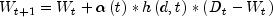where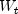represents the node weights,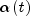is the monotonically decreasing learning coefficient function,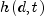is the neighborhood function, d is the lattice distance between the node and the BMU, and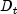is the input vector.

The monotonically decreasing learning coefficient functionis a scalar factor that defines the size of the update correction. The value ofdecreases with the step index t.

The neighborhood functiondepends on the lattice distance d between the node and the BMU, and represents the strength of the coupling between the node and BMU. In the simplest form, the value ofis 1 for all nodes closest to the BMU and 0 for others, but a Gaussian function is also commonly used. Regardless of the functional form, the neighborhood function shrinks with time (Hollm�n, 15.2.1996). Early on, when the neighborhood is broad, the self-organizing takes place on the global scale. When the neighborhood has shrunk to just a couple of nodes, the weights converge to local estimates.

Note that in a rectangular grid, the BMU has four closest nodes for the Von Neumann neighborhood type, or eight closest nodes for the Moore neighborhood type. In a hexagonal grid, the BMU has six closest nodes.

During training, this process is repeated for a number of iterations on all input vectors.

During forecasting, the node with the shortest Euclidean distance is the winning node, and its (i, j) index is the output.

Example, Serialized Form
• ### Field Summary

Fields
Modifier and Type Field and Description
`static int` `GRID_HEXAGONAL`
Indicates a hexagonal grid.
`static int` `GRID_RECTANGULAR`
Indicates a rectangular grid.
`static int` `TYPE_MOORE`
Indicates a Moore neighborhood type.
`static int` `TYPE_VON_NEUMANN`
Indicates a Von Neumann neighborhood type.
• ### Constructor Summary

Constructors
Constructor and Description
```KohonenSOM(int dim, int nrow, int ncol)```
Constructor for a `KohonenSOM` object.
• ### Method Summary

Methods
Modifier and Type Method and Description
`int[]` `forecast(double[] input)`
Returns a forecast computed using the `KohonenSOM` object.
`int[][]` `forecast(double[][] input)`
Returns forecasts computed using the `KohonenSOM` object.
`int` `getDimension()`
Returns the number of weights for each node.
`int` `getGridType()`
Returns the grid type.
`int` `getNeighborhoodType()`
Returns the neighborhood type for the rectangular grid.
`int` `getNumberOfColumns()`
Returns the number of columns of the node grid.
`int` `getNumberOfRows()`
Returns the number of rows of the node grid.
`double[][][]` `getWeights()`
Returns the weights of the nodes.
`double[]` ```getWeights(int i, int j)```
Returns the weights of the node at (i, j) in the node grid.
`boolean` `isWrapAround()`
Returns whether the opposite edges are connected or not.
`void` `setGridType(int type)`
Sets the grid type.
`void` `setNeighborhoodType(int type)`
Sets the neighborhood type.
`void` `setWeights()`
Sets the weights of the nodes using random numbers.
`void` `setWeights(double[][][] weights)`
Sets the weights of the nodes.
`void` ```setWeights(int i, int j, double[] weights)```
Sets the weights of the node at (i, j) in the node grid.
`void` `setWeights(Random random)`
Sets the weights of the nodes using a `Random` object.
`void` `wrapAround()`
Sets a flag to indicate the map should wrap around or connect opposite edges.
• ### Methods inherited from class java.lang.Object

`clone, equals, finalize, getClass, hashCode, notify, notifyAll, toString, wait, wait, wait`
• ### Field Detail

• #### GRID_HEXAGONAL

`public static final int GRID_HEXAGONAL`
Indicates a hexagonal grid.
Constant Field Values
• #### GRID_RECTANGULAR

`public static final int GRID_RECTANGULAR`
Indicates a rectangular grid.
Constant Field Values
• #### TYPE_MOORE

`public static final int TYPE_MOORE`
Indicates a Moore neighborhood type.
Constant Field Values
• #### TYPE_VON_NEUMANN

`public static final int TYPE_VON_NEUMANN`
Indicates a Von Neumann neighborhood type.
Constant Field Values
• ### Constructor Detail

• #### KohonenSOM

```public KohonenSOM(int dim,
int nrow,
int ncol)```
Constructor for a `KohonenSOM` object.
Parameters:
`dim` - An `int` scalar containing the number of weights for each node in the node grid. `dim` must be greater than zero.
`nrow` - An `int` scalar containing the number of rows in the node grid. `nrow` must be greater than zero.
`ncol` - An `int` scalar containing the number of columns in the node grid. `ncol` must be greater than zero.
• ### Method Detail

• #### forecast

`public int[] forecast(double[] input)`
Returns a forecast computed using the `KohonenSOM` object.
Parameters:
`input` - A `double` array containing the input data. `input.length` must be equal to `dim`.
Returns:
An `int` array of length 2 containing the (i, j) index of the output node.
• #### forecast

`public int[][] forecast(double[][] input)`
Returns forecasts computed using the `KohonenSOM` object.
Parameters:
`input` - A `double` matrix containing `input.length` observations of data. `input[i].length` must be equal to `dim`.
Returns:
An `int` matrix containing the output indices of the nodes. The i-th row contains the (i, j) index of the output node for `input[i]`.
• #### getDimension

`public int getDimension()`
Returns the number of weights for each node.
Returns:
An `int` scalar containing the number of weights for each node.
• #### getGridType

`public int getGridType()`
Returns the grid type.
Returns:
An `int` scalar containing the grid type. The return value is either `KohonenSOM.GRID_RECTANGULAR` or `KohonenSOM.GRID_HEXAGONAL`
• #### getNeighborhoodType

`public int getNeighborhoodType()`
Returns the neighborhood type for the rectangular grid.
Returns:
An `int` scalar containing the neighborhood type. The return value is either `KohonenSOM.TYPE_VON_NEUMANN` or `KohonenSOM.TYPE_MOORE`
• #### getNumberOfColumns

`public int getNumberOfColumns()`
Returns the number of columns of the node grid.
Returns:
An `int` scalar containing the number of columns of the node grid.
• #### getNumberOfRows

`public int getNumberOfRows()`
Returns the number of rows of the node grid.
Returns:
An `int` scalar containing the number of rows of the node grid.
• #### getWeights

`public double[][][] getWeights()`
Returns the weights of the nodes.
Returns:
An `nrow` by `ncol` matrix of double arrays containing the weights of the nodes.
• #### getWeights

```public double[] getWeights(int i,
int j)```
Returns the weights of the node at (i, j) in the node grid.
Parameters:
`i` - An `int` scalar containing the row index of the node in the node grid, where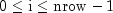.
`j` - An `int` scalar containing the column index of the node in the node grid, where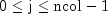.
Returns:
A `double` array containing the weights of the node at (i, j) in the node grid.
• #### isWrapAround

`public boolean isWrapAround()`
Returns whether the opposite edges are connected or not.
Returns:
A `boolean` indicating whether or not the opposite edges are connected. It is true if the opposite edges are connected. Otherwise, it is false.
• #### setGridType

`public void setGridType(int type)`
Sets the grid type.
Parameters:
`type` - An `int` scalar containing the grid type, rectangular (`KohonenSOM.GRID_RECTANGULAR`) or hexagonal (`KohonenSOM.GRID_HEXAGONAL`).

Default: `type` = `GRID_RECTANGULAR`.

 `type` Description `GRID_RECTANGULAR` Use a rectangular grid (`type` = 0). `GRID_HEXAGONAL` Use a hexagonal grid (`type` = 1).
• #### setNeighborhoodType

`public void setNeighborhoodType(int type)`
Sets the neighborhood type.
Parameters:
`type` - An `int` scalar containing the neighborhood type, Von Neumann (`KohonenSOM.TYPE_VON_NEUMANN`) or Moore (`KohonenSOM.TYPE_MOORE`). This method is ignored for a hexagonal grid.

Default: `type` = `TYPE_VON_NEUMANN`.

 `type` Description `TYPE_VON_NEUMANN` Use the Von Neumann (`type` = 0) neighborhood type. `TYPE_MOORE` Use the Moore (`type` = 1) neighborhood type.
• #### setWeights

`public void setWeights()`
Sets the weights of the nodes using random numbers. The weights are in [0.0, 1.0].
• #### setWeights

`public void setWeights(double[][][] weights)`
Sets the weights of the nodes.
Parameters:
`weights` - An `nrow` by `ncol` matrix of double arrays containing the weights of the nodes. `weights[i][j].length` must be equal to `dim`.
• #### setWeights

```public void setWeights(int i,
int j,
double[] weights)```
Sets the weights of the node at (i, j) in the node grid.
Parameters:
`i` - An `int` scalar containing the row index of the node in the node grid, where.
`j` - An `int` scalar containing the column index of the node in the node grid, where.
`weights` - A `double` array containing the weights. `weights.length` must be equal to `dim`.
• #### setWeights

`public void setWeights(Random random)`
Sets the weights of the nodes using a `Random` object. The weights are generated using the `Random.nextDouble` method.
Parameters:
`random` - A `Random` object used to generate random numbers for the nodes.
• #### wrapAround

`public void wrapAround()`
Sets a flag to indicate the map should wrap around or connect opposite edges. A hexagonal grid must have an even number of rows to wrap around. By default, opposite edges are not connected.
JMSLTM Numerical Library 7.2.0